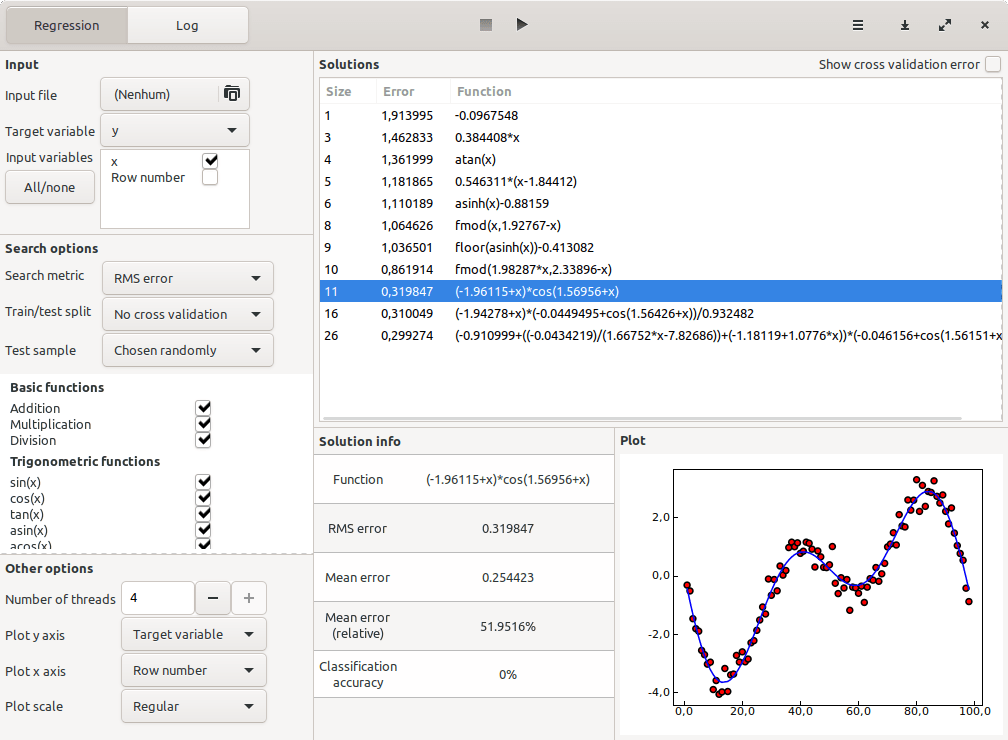# Machine learning black box models: some alternatives

In this article, we will discuss a very basic question regarding machine learning: is every model a black box? Certainly most methods seem to be, but as we will see, there are very interesting exceptions to this.

### What is a black box method?

A method is said to be a black box when it performs complicated computations under the hood that cannot be clearly explained and understood. Data is fed into the model, internal transformations are performed on this data and an output is given, but these transformations are such that basic questions cannot be answered in a straightforward way:

• Which of the input variables contributed the most to generating the output?
• Exactly what features did the model derive from the input data?
• How does the output change as a function of one of the variables?

Not only are black box models hard to understand, they are also hard to move around: since complicated data structures are necessary for the relevant computations, they cannot be readily translated to different programming languages.

### Can there be machine learning without black boxes?

The answer to that question is yes. In the simplest case, a machine learning model can be a linear regression and consist of a line defined by an explicit algebraic equation. This is not a black box method, since it is clear how the variables are being used to compute an output.

But linear models are quite limited and cannot perform the same kinds of tasks that neural networks do, for example. So a more interesting question is: is there a machine learning method capable of finding nonlinear patterns in an explicit and understandable way?

It turns out that such method exists, and is called symbolic regression.

### Symbolic regression as an alternative

The idea of symbolic regression is to find explicit mathematical formulas that connect input variables to an output, while trying to keep those formulas as simple as possible. The resulting models end up being explicit equations that can be written on a sheet of paper, making it apparent how the input variables are being used despite the presence of nonlinear computations.

To give a clearer picture, consider some models found by TuringBot, a symbolic regression software for PC:Symbolic models found by the TuringBot symbolic regression software.

In the “Solutions” box above, a typical result of a symbolic regression optimization can be seen. A set of formulas of increasing complexity was found, with more complex formulas only being shown if they perform better than all simpler alternatives. A nonlinearity in the input dataset was successfully recovered through the use of nonlinear base functions like cos(x), atan(x) and multiplication.

Symbolic regression is a very general technique: although the most obvious use case is to solve regression problems, it can also be used to solve classification problems by representing categorical variables as different integer numbers, and running the optimization with classification accuracy as the search metric instead of RMS error. Both of these options are available in TuringBot.

### Conclusion

In this article, we have seen that despite most machine learning methods indeed being black boxes, not all of them are. A simple counterexample are linear models, which are explicit and hence not black boxes. More interestingly, we have seen how symbolic regression is capable of solving machine learning tasks where nonlinear patterns are present, generating models that are mathematical equations that can be analyzed and interpreted.